# Theoretical Metal Weight Calculation Formula (30 Types of Metals)

If you’re working in the metalworking industry, even you’re engineers, you will try to find one calculator to help you calculate the weight of various metals and steels including ms plate, gi sheet, structural steel, ms angle, mild steel, steel bar, square tube, angle, aluminum etc.

## Theoretical Metal Weight Calculator

Below is the calculator for you to calculate the weight of various metals. What you need to do is calculate the sectional area and find the metal density.

For the metal density, you can also refer to:

From the above two posts, you will find the density of various metals and alloys.

However, sometimes you may want to learn the weight calculation formula and keep it in your mind, here is it:

Weight (kg) = Sectional Area (mm2) × Length (m) × Density (ρ, g/cm3)× 1/1000

In order to help you quickly find the metal weight formula, I made infographic which is not only practical but also beautiful. (To the bottom)

P.S: Among all of the metal weight calculation formula in the following part, there are two kinds of metals that are not mentioned, which are I beam steel and H beam steel.

If you want to know the difference between H beam steel and I beam steel as well as the methods to calculate the weight of H beam steel and I beam steel, you will get the answers from the following two quick links:

## Theoretical Steel Weight Chart

If you want to get the weight of various metals from the chart, you can refer to the following page:### Steel Plate

• Formula: length(m)×width(m)×thickness(mm)×7.85
• E.g: 6m (length)×1.51m(width)×9.75mm (thickness)
• Calculation: 6×1.51×9.75×7.85=693.43kg### Steel Tube

• Formula: (OD-wall thickness)×wall thickness(mm)×length(m)×0.02466
• E.g: 114mm(OD)×4mm(wall thickness)×6m(length)
• Calculation: (114-4)×4×6×0.02466=65.102kg### Steel Rod

• Formula: dia.(mm)×dia.(mm)×length(m)×0.00617
• E.g: Φ20mm (dia.)×6m(length)
• Calculation: 20×20×6×0.00617=14.808kg### Square Steel

• Formula: edge width(mm)×edge width(mm)×length(m)×0.00785
• E.g: 50mm(edge width)×6m(length)
• Calculation: 50×50×6×0.00785=117.75(kg)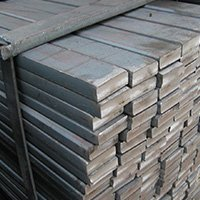### Flat Steel

• Formula: edge width(mm)×thickness(mm)×length(m)×0.00785
• E.g: 50mm(edge width)×5.0mm(thickness)×6m(length)
• Calculation: 50×5×6×0.00785=11.7.75(kg)### Hexagonal Steel

• Formula: edge-to-edge dia.×edge-to-edge dia.× length(m)×0.0068
• E.g: 50mm(dia.)×6m (length)
• Calculation: 50×50×6×0.0068=102(kg)### Rebar

• Formula: dia.mm×dia.mm×length(m)×0.00617
• E.g: Φ20mm(dia.)×12m(length)
• Calculation: 20×20×12×0.00617=29.616kg### Flat Steel Tube

• Formula: (edge length+edge width)×2×thickness×length(m)×0.00785
• E.g: 100mm×50mm×5mm(thickness)×6m (length)
• Calculation: (100+50)×2×5×6×0.00785=70.65kg### Rectangular Steel Tube

• Formula: Edge width(mm)×4×thickness×length(m)×0.00785
• E.g: 50mm×5mm (thickness)×6m(length)
• Calculation: 50×4×5×6×0.00785=47.1kg### Equal-leg Angle Steel

• Formula: (edge width×2-thickness)×thickness×length(m)×0.00785
• E.g: 50mm×50mm×5(thickness)×6m(length)
• Calculation: (50×2-5)×5×6×0.00785=22.37kg### Unequal-leg Angle Steel

• Formula: (edge width+edge width-thickness)×thickness×length(m)×0.0076
• E.g: 100mm×80mm×8(thickness)×6m(length)
• Calculation: (100+80-8)×8×6×0.0076=62.746kg### Brass Tube

• Formula: (OD-wall thickness)×thickness(mm)×length(m)×0.0267### Copper Tube

• Formula: (OD-wall thickness)×thickness(mm)×length(m)×0.02796### Aluminum Embossed Sheet

• Formula: length(m)×width(mm)×thickness (mm)×0.00296### Brass Tube

• Formula: thickness(mm)×(O.D-thickness)×length(m)×0.0267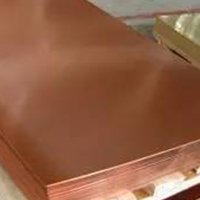### Copper Sheet

• Formula: length(m)×width(mm)×thickness(mm)×0.0089### Zinc Plate

• Formula: length(m)×width(mm)×thickness(mm)×0.0072• Formula: length(m)×width(mm)×thickness(mm)×0.01137### Octagonal Steel

• Formula: length(m)×across width(mm)×across width(mm)×0.0065### Copper Rod

• Formula: dia.(mm)×dia.(mm)×length(m)×0.00698### Brass Rod

• Formula: dia.(mm)×dia.(mm)×length(m)×0.00668### Aluminum Rod

• Formula: dia.(mm)×dia.(mm)×length(m)×0.0022### Square Copper Rod

• Formula: width(mm)×width(mm)×length(m)×0.0089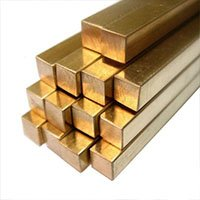### Square Brass Rod

• Formula: width(mm)×width(mm)×length(m)×0.0085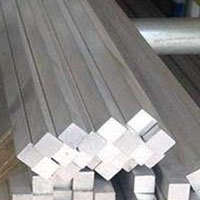### Square Aluminum Rod

• Formula: width(mm)×width(mm)×length(m)×0.0028### Hexagonal Copper Rod

• Formula: across width(mm)×across width(mm)×length(m)×0.0077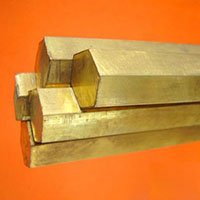### Hexagonal Brass Rod

• Formula: width(mm)×across width(mm)×length(m)×0.00736### Hexagonal Aluminum Rod

• Formula: across width(mm)×across width(mm)×length(m)×0.00242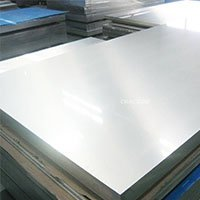### Aluminum-Plate.jpg

• Formula: thickness(mm)×width(mm)×length(m)×0.00171### Aluminum Tube

• Formula: thickness(mm)×(O.D(mm)-thickness(mm))×length(m)×0.00879

### 411 thoughts on “Theoretical Metal Weight Calculation Formula (30 Types of Metals)”

1. Santosh S Datar

Sir,
Pl explain hoe does the density 0.02466 arrived for calculation of weight in case of Tubes.

2. this very interesting for the formula to calculate any for steel , it so very helful

3. Valuable details, Good effort.
Please send me the PDF file.

4. I HAVE SEEN MANY SITES WHO PROVIDE YOU THE RESULT BUY DOES NOT SHARE THE CALCULATION. AS A SHOP FLOOR PERSON WE CAN NOT BE ONLINE ALL THE TIME. THANKS FOR SHARING VALUABLE INFORMATION AND PLEASE DO SHARE THE PDF VERSION ALSO.YOUR WORK IS ADMIRABLE. GOOD SITE

DON’T MISS OUT!